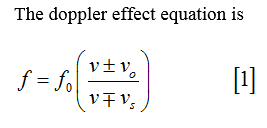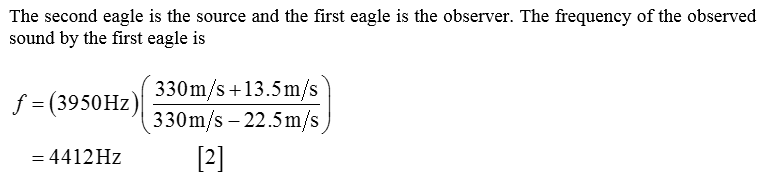# Two eagles fly directly toward one another, the first at 13.5 m/s and the second at 22.5 m/s. Both screech, the first one emitting a frequency of 2950 Hz and the second one emitting a frequency of 3950 Hz.What frequency of sound, in hertz, does the first eagle hear from the second if the speed of sound is 330 m/s? What frequency of sound, in hertz, does the second eagle hear from the first if the speed of sound is 330 m/s?

Question
10 views

Two eagles fly directly toward one another, the first at 13.5 m/s and the second at 22.5 m/s. Both screech, the first one emitting a frequency of 2950 Hz and the second one emitting a frequency of 3950 Hz.

What frequency of sound, in hertz, does the first eagle hear from the second if the speed of sound is 330 m/s?

What frequency of sound, in hertz, does the second eagle hear from the first if the speed of sound is 330 m/s?

check_circle

Step 1Step 2...

### Want to see the full answer?

See Solution

#### Want to see this answer and more?

Solutions are written by subject experts who are available 24/7. Questions are typically answered within 1 hour.*

See Solution
*Response times may vary by subject and question.
Tagged in